# Setup for 4 Player a Game of Food Chain Magnate

Originally generated on 1/11/2019 12:13:47 PM
A direct link to this setup is http://www.VentersConsulting.com/BoardgameHelpers/FoodChainMagnateGenerator.aspx?LoadGame=\$2a\$04\$6is0z6VOUyvBnse.pbZ4ZO

## Player Setup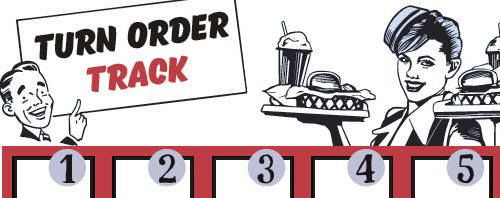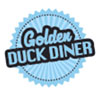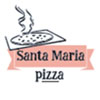## Other Setup

Bank starts with \$200.00
Remove these Billboards: #16
Number of 1x Employee cards used = 2

## Map (Balanced)

Click here to view Map Tile Lettering Key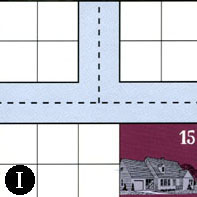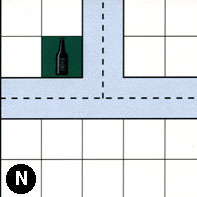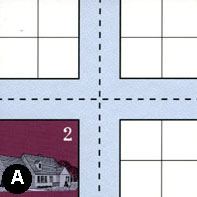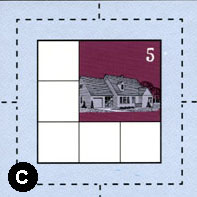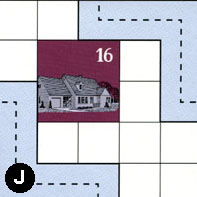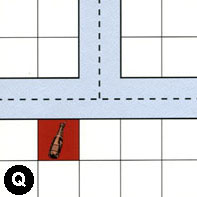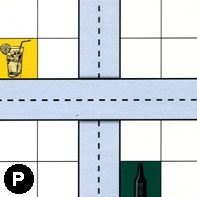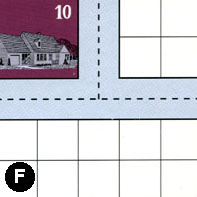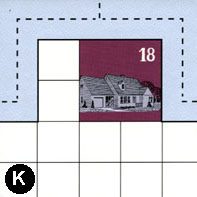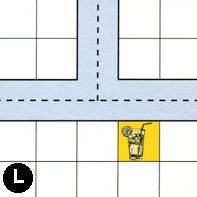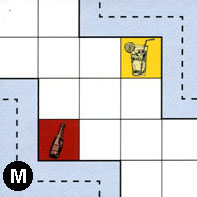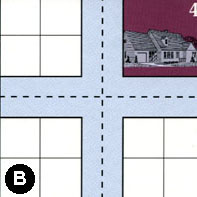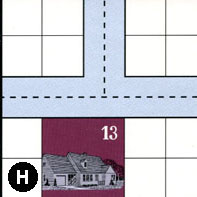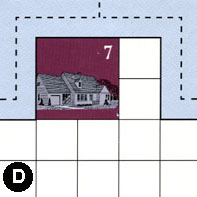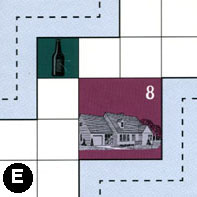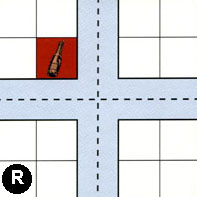### Map Stats

Map Option = Balanced
Balance Issues = Not enough neighborhoods. Not enough large neighborhoods. Not enough empty space in neighborhoods.
Total Number of Tiles = 16
Total Number of Starting Houses = 10
Total Number of Beer Spots = 3
Total Number of Soda Spots = 3
Total Number of Lemonade Spots = 3
Total Number of Drink Spots = 9
Number of independent path systems = 3
Number of independent neighborhoods = 14

Path System 1
Size = Medium
Number of Tiles = 13
Number of Paths = 44
Contains Loop(s) = No
Number of Starting Houses = 7
Starting Houses Pct of Map (%) = 70
Number of Beer Spots = 2
Number of Soda Spots = 3
Number of Lemonade Spots = 3
Number of Drink Spots = 8
Drink Spots Pct of Map (%) = 89
Tile Ids = A, B, C, E, F, I, J, L, M, N, P, Q, R
Paths = IWN, NWN, NNE, AWS, AWN, CWN, CNE, CWE, ANE, PWE, MEN, LWE, LWN, QWN, JEN, IWE, INE, NWE, ANS, CWS, FWN, FNE, BWN, BNE, RWN, EWN, MWS, BWS, FWE, CNS, CES, BWE, RNE, RWE, RWS, RNS, BES, BNS, RES, AES, AWE, QNE, QWE, LNE What do these mean?
Starting House Ids = 0, 2, 4, 5, 10, 15, 16
Path System 2
Size = Medium
Number of Tiles = 5
Number of Paths = 11
Contains Loop(s) = No
Number of Starting Houses = 5
Starting Houses Pct of Map (%) = 50
Number of Beer Spots = 0
Number of Soda Spots = 0
Number of Lemonade Spots = 0
Number of Drink Spots = 0
Drink Spots Pct of Map (%) = 0
Tile Ids = D, E, H, J, K
Paths = JWS, KWE, HWN, HWE, DWE, EES, DWN, DNE, HNE, KWN, KNE What do these mean?
Starting House Ids = 7, 8, 13, 16, 18
Path System 3
Size = Tiny
Number of Tiles = 1
Number of Paths = 1
Contains Loop(s) = No
Number of Starting Houses = 1
Starting Houses Pct of Map (%) = 10
Number of Beer Spots = 1
Number of Soda Spots = 0
Number of Lemonade Spots = 0
Number of Drink Spots = 1
Drink Spots Pct of Map (%) = 11
Tile Ids = P
Paths = PNS What do these mean?
Starting House Ids = 0

Neighborhood 1
Total Size = Large
Number of Total Spaces = 49
Number of Empty Spaces (for new houses & gardens) = 37
Number of Beer Spots = 0
Number of Soda Spots = 0
Number of Lemonade Spots = 0
Number of Drink Spots = 0
Number of Starting Houses = 3
Starting Houses Pct of Map (%) = 30
Tile Ids = I, J, K, L, S
Starting House Ids = 15, 16, 18
Neighborhood 2
Total Size = Medium
Number of Total Spaces = 8
Number of Empty Spaces (for new houses & gardens) = 8
Number of Beer Spots = 0
Number of Soda Spots = 0
Number of Lemonade Spots = 0
Number of Drink Spots = 0
Number of Starting Houses = 0
Starting Houses Pct of Map (%) = 0
Tile Ids = I, N
Neighborhood 3
Total Size = Medium
Number of Total Spaces = 26
Number of Empty Spaces (for new houses & gardens) = 22
Number of Beer Spots = 0
Number of Soda Spots = 0
Number of Lemonade Spots = 0
Number of Drink Spots = 0
Number of Starting Houses = 1
Starting Houses Pct of Map (%) = 10
Tile Ids = A, N, P, S
Starting House Ids = 2
Neighborhood 4
Total Size = Small
Number of Total Spaces = 4
Number of Empty Spaces (for new houses & gardens) = 4
Number of Beer Spots = 0
Number of Soda Spots = 0
Number of Lemonade Spots = 0
Number of Drink Spots = 0
Number of Starting Houses = 0
Starting Houses Pct of Map (%) = 0
Tile Ids = A
Neighborhood 5
Total Size = Medium
Number of Total Spaces = 9
Number of Empty Spaces (for new houses & gardens) = 5
Number of Beer Spots = 0
Number of Soda Spots = 0
Number of Lemonade Spots = 0
Number of Drink Spots = 0
Number of Starting Houses = 1
Starting Houses Pct of Map (%) = 10
Tile Ids = C
Starting House Ids = 5
Neighborhood 6
Total Size = Medium
Number of Total Spaces = 12
Number of Empty Spaces (for new houses & gardens) = 10
Number of Beer Spots = 1
Number of Soda Spots = 1
Number of Lemonade Spots = 0
Number of Drink Spots = 2
Number of Starting Houses = 0
Starting Houses Pct of Map (%) = 0
Tile Ids = I, N, S
Neighborhood 7
Total Size = Medium
Number of Total Spaces = 12
Number of Empty Spaces (for new houses & gardens) = 11
Number of Beer Spots = 0
Number of Soda Spots = 0
Number of Lemonade Spots = 1
Number of Drink Spots = 1
Number of Starting Houses = 0
Starting Houses Pct of Map (%) = 0
Tile Ids = A, F, P
Neighborhood 8
Total Size = Medium
Number of Total Spaces = 14
Number of Empty Spaces (for new houses & gardens) = 14
Number of Beer Spots = 0
Number of Soda Spots = 0
Number of Lemonade Spots = 0
Number of Drink Spots = 0
Number of Starting Houses = 0
Starting Houses Pct of Map (%) = 0
Tile Ids = B, F
Neighborhood 9
Total Size = Medium
Number of Total Spaces = 12
Number of Empty Spaces (for new houses & gardens) = 10
Number of Beer Spots = 2
Number of Soda Spots = 0
Number of Lemonade Spots = 0
Number of Drink Spots = 2
Number of Starting Houses = 0
Starting Houses Pct of Map (%) = 0
Tile Ids = L, P, S
Neighborhood 10
Total Size = Large
Number of Total Spaces = 86
Number of Empty Spaces (for new houses & gardens) = 66
Number of Beer Spots = 1
Number of Soda Spots = 1
Number of Lemonade Spots = 2
Number of Drink Spots = 4
Number of Starting Houses = 4
Starting Houses Pct of Map (%) = 40
Tile Ids = B, D, E, F, H, L, M, P, R
Starting House Ids = 7, 8, 10, 13
Neighborhood 11
Total Size = Medium
Number of Total Spaces = 8
Number of Empty Spaces (for new houses & gardens) = 7
Number of Beer Spots = 0
Number of Soda Spots = 1
Number of Lemonade Spots = 0
Number of Drink Spots = 1
Number of Starting Houses = 0
Starting Houses Pct of Map (%) = 0
Tile Ids = B, R
Neighborhood 12
Total Size = Medium
Number of Total Spaces = 8
Number of Empty Spaces (for new houses & gardens) = 4
Number of Beer Spots = 0
Number of Soda Spots = 0
Number of Lemonade Spots = 0
Number of Drink Spots = 0
Number of Starting Houses = 1
Starting Houses Pct of Map (%) = 10
Tile Ids = B, R
Starting House Ids = 4
Neighborhood 13
Total Size = Small
Number of Total Spaces = 4
Number of Empty Spaces (for new houses & gardens) = 4
Number of Beer Spots = 0
Number of Soda Spots = 0
Number of Lemonade Spots = 0
Number of Drink Spots = 0
Number of Starting Houses = 0
Starting Houses Pct of Map (%) = 0
Tile Ids = H
Neighborhood 14
Total Size = Small
Number of Total Spaces = 4
Number of Empty Spaces (for new houses & gardens) = 4
Number of Beer Spots = 0
Number of Soda Spots = 0
Number of Lemonade Spots = 0
Number of Drink Spots = 0
Number of Starting Houses = 0
Starting Houses Pct of Map (%) = 0
Tile Ids = R

v8.3.2